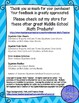# Distributive Property and Like Terms on Both Sides Equations Activity BundleSubject
Resource Type
File Type

Zip

(17 MB|68 pages)
Standards
5 Products in this Bundle
5 products
1. This set of 32 equations task cards with combining like terms on both sides is a great step to getting students to solve equations by combining like terms and using the distributive property. This scaffolded set of equations involves students combining like terms on both sides of the equation befor
2. This set of 32 equation task cards with distributive property on both sides is a perfect step in getting students to solve equations with both combining like terms and distributive property. In every equation in the set, students must use the distributive property to simplify the equation before so
3. These 8 task cards/posters give students the opportunity to assess, explain, and correct common misconceptions when solving equations with combining like terms and distributive property. A great end of the unit review for 8th graders! Distributive property on both sides is used in the first 3 cards
4. This independent review activity allows students the opportunity to solve equations with distributive property and combining like terms on BOTH SIDES of the equation and gives YOU the opportunity to differentiate! Option One gives students 12 equations to solve and color the corresponding spot on a
5. This independent review activity allows students the opportunity to solve equations with distributive property and combining like terms on BOTH SIDES of EVERY equation and gives YOU the opportunity to differentiate! Option One gives students 12 equations to solve and color the corresponding spot on
• Bundle Description
• Standards

5 PRODUCTS FOR ONE LOW PRICE!!! Looking for ways for your students to practice solving equations with distributive property and combining like terms on both sides of the equation? This is the product for you! The 64 task cards offer practice solving equations with combining like terms and distributive property on both sides of the equation separately. The 4 color sheets give you option to combine distributive property and like terms in the same equation or to keep them separate. The 8 error analysis problems combine all of the equation types and give your students the opportunity to demonstrate their high order thinking!

Products included in this bundle:

Combining Like Terms on Both Sides Equations Task Cards

Distributive Property on Both Sides Equation Task Cards

Distributive Property and Like Terms on Both Sides Equations Color Sheets

Distributive Property and Like Terms Combined Equations Color Sheets

Error Analysis Equations with Distributive Property and Combining Like Terms

Check out these other equation task card products!

Distributive Property and Like Terms Equations Task Cards

Equations Task Cards with Variables on Both Sides

Check out my color sheets for great equations review!

Distributive Property and Like Terms Equations Color Sheets

Variables on Both Sides Equations Color Sheets

Check out these other equations bundles!

Equations Color Sheets Bundle

Variables on Both Sides Equations Activity Bundle

Check out these other awesome products!

Error Analysis Equations Cards FREEBIE

Number of Solutions Equations Notebook Discovery Activity

Solve linear equations with rational number coefficients, including equations whose solutions require expanding expressions using the distributive property and collecting like terms.
Solve linear equations in one variable.
Total Pages
68 pages
Included
Teaching Duration
3 days
Report this Resource to TpT
Reported resources will be reviewed by our team. Report this resource to let us know if this resource violates TpT’s content guidelines.